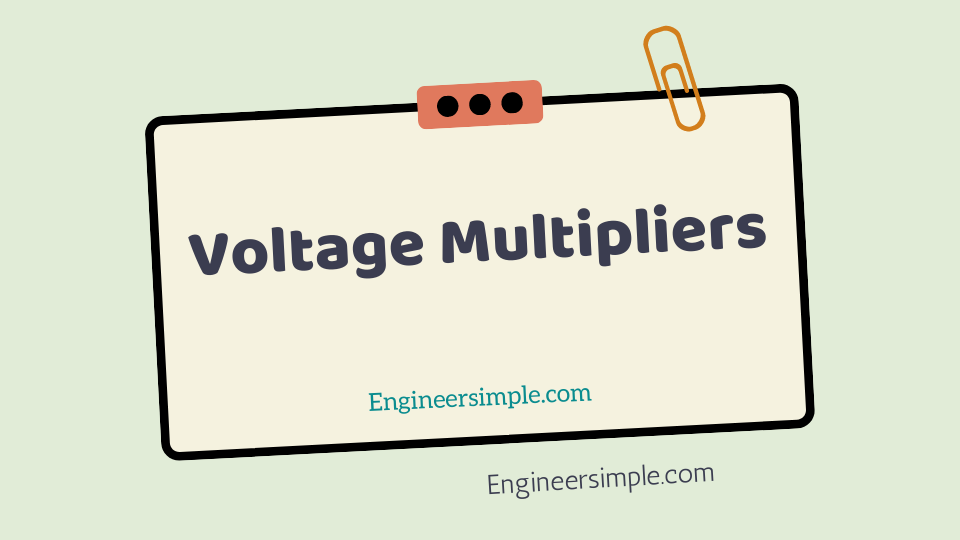Voltage Multipliers - Engineer Simple

#Voltage Multipliers-With a diode, we can build a rectifier to produce a d.c. voltage that is nearly equal to the
peak value of a.c. voltage. We can also use diodes and capacitors to build a circuit that
will provide a d.c output that is multiple of the peak input a.c. voltage.

Such a circuit is
call a voltage multiplier. For example, a voltage doubler will provide a d.c. output that
is twice the peak input a.c. voltage, a voltage tripler will provide a d.c. output that is
three times the peak input a.c voltage and so on.

While voltage multipliers provide d.c. output that is much greater than the peak
input a.c. voltage, there is no power amplification and law of conservation of energy
holds good. When a voltage multiplier increases the peak input voltage by a factor n,

the peak input current is decreased by approximately the same factor. Thus the actual
power output from a voltage multiplier will never be greater than the input power. In
fact, there are losses in the circuit e.g. in diodes, capacitors etc.) so that the output
power will actually be less than the input power.

## Half-wave Voltage Doubler

A half-wave voltage doubler consists of two diodes and two capacitors connected in a
manner as shown in Fig.1. It will be shown that if the peak input a.c. voltage is V s(pk)
, the d.c. output voltage will be 2 V s(pk) provided the diodes are ideal (this assuption is
fairly reasonable).

The basic idea in a voltage multiplier is to charge each capacitor to
the peak input a.c. voltage and to arrange the capacitors so that their stored voltages

## Half-wave Voltage Doublers

A half-wave voltage doubler consists of two diodes and two capacitors connected in a
manner as shown in Fig.1. It will be shown that if the peak input a.c. voltage is V s(pk)
, the d.c. output voltage will be 2 V s(pk) provided the diodes are ideal (this assuption is
fairly reasonable).

The basic idea in a voltage multiplier is to charge each capacitor to
the peak input a.c. voltage and to arrange the capacitors so that their stored voltages

### Circuit action

#### We now discuss the working of half-wave voltage doublers:

(i) During the negative half-cycle of a.c. input voltage [See Fig.2 (a)],
diode D 1 is forward biased and diode D 2 is reverse biased [See See Fig.
2 (a)].

Therefore, diode D 1 can be represent by a short and diode D 2
as an open.

The equivalent circuit then becomes as shown in Fig. 2 (b).
As you can see [See Fig.2 (B)], C 1 will charge until voltage across it becomes
equal to peak value of source voltage [V s(pk) ]. At the same time, C 2 will be in the process
of discharging through the load R L (The source of this charge on C 2 will be explain in
a moment).

##### Note that in all figures electron flow is show.

(ii) When the polarity of the input a.c. voltage reverses (i.e, during positive
half-cycle), the circuit conditions become as shown in Fig. 2.
Referring to Fig.2, it is easy to see that C 1 (charged to V s(pk) ) and the
source voltage (V s ) now act as series-aiding voltage sources. Thus C 2 will be charge
to the sum of the series peak voltage i.e. 2 V s(pk).

(iii)When V s returns to its original polarity (i.e. negative half-cycle), D 2 is again
turned off (i.e, reverse biased). With D 2 turned off, the only discharge path for C 2 is
through the load resistance R L . The time constant (=R L C 2 ) of this circuit is so adjust
that C 2 has little time to lose any of its charge before the input polarity reverses again.
During the positive half-cycle, D 2 is turned on and C 2 recharges until voltage across it is
again equal to 2 V s(pk).

D.C. output voltage, V dc = 2 V s(pk)
Since C 2 barely discharges between input cycles, the output waveform of the
half-wave voltage doublers closely resembles that of a filtered half-wave rectifier. Fig.
2 shows the input and output waveforms for a half-wave voltage doublers.
The voltage multipliers have the disadvantage of poor voltage regulation. This
means that c.c. output voltage drops considerably as the load current increases. Large
filter capacitors are needed to help maintain the output voltage.